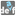• # I don't get what should come out of "apb", "asrqb"

## Question related to missionDetermine the Order

As title says,

a < p < b

a < s < r < q < b

so we are missing information on the relation of p to q,r,s

so the result could be either

p < s < r < q

s < p < r < q

s < r < p < q

s < r < q < p

Natural ordering does not help here as we are certain about the position of "srq"!17